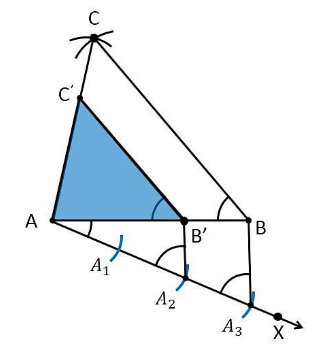Guru

# Construct a triangle of sides 4 cm, 5 cm and 6 cm and then a triangle similar to it whose sides are 2/3 of the corresponding sides of the first triangle. Q.2

• 0

What is the best way to solve the problem of question no.2 of exercise 11.1 of constructions of question no.2, please give me the best and easy way to solve this problem Construct a triangle of sides 4 cm, 5 cm and 6 cm and then a triangle similar to it whose sides are 2/3 of the corresponding sides of the first triangle.

Share

1. Construction Procedure:

1. Draw a line segment AB which measures 4 cm, i.e., AB = 4 cm.

2. Take the point A as centre, and draw an arc of radius 5 cm.

3. Similarly, take the point B as its centre, and draw an arc of radius 6 cm.

4. The arcs drawn will intersect each other at point C.

5. Now, we obtained AC = 5 cm and BC = 6 cm and therefore ΔABC is the required triangle.

6. Draw a ray AX which makes an acute angle with the line segment AB on the opposite side of vertex C.

7. Locate 3 points such as A1, A2, A3 (as 3 is greater between 2 and 3) on line AX such that it becomes AA1= A1A2 = A2A3.

8. Join the point BA3 and draw a line through A2which is parallel to the line BA3 that intersect AB at point B’.

9. Through the point B’, draw a line parallel to the line BC that intersect the line AC at C’.

10. Therefore, ΔAB’C’ is the required triangle.Justification:

The construction of the given problem can be justified by proving that

AB’ = (2/3)AB

B’C’ = (2/3)BC

AC’= (2/3)AC

From the construction, we get B’C’ || BC

∴ ∠AB’C’ = ∠ABC (Corresponding angles)

In ΔAB’C’ and ΔABC,

∠ABC = ∠AB’C (Proved above)

∠BAC = ∠B’AC’ (Common)

∴ ΔAB’C’ ∼ ΔABC (From AA similarity criterion)

Therefore, AB’/AB = B’C’/BC= AC’/AC …. (1)

In ΔAAB’ and ΔAAB,

∠A2AB’ =∠A3AB (Common)

From the corresponding angles, we get,

∠AA2B’ =∠AA3B

Therefore, from the AA similarity criterion, we obtain

ΔAA2B’ and AA3B

So, AB’/AB = AA2/AA3

Therefore, AB’/AB = 2/3 ……. (2)

From the equations (1) and (2), we get

AB’/AB=B’C’/BC = AC’/ AC = 2/3

This can be written as

AB’ = (2/3)AB

B’C’ = (2/3)BC

AC’= (2/3)AC

Hence, justified.

• 0